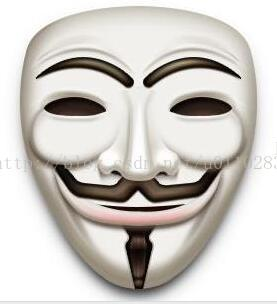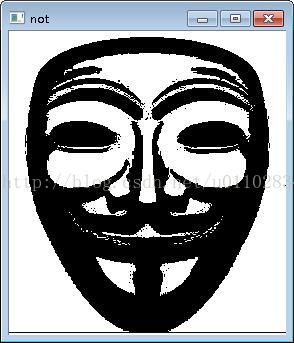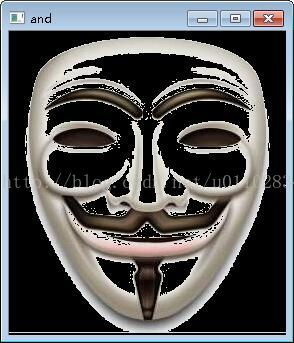MrLee8月前 607

# 1.图像基本运算

```void add(InputArray src1, InputArray src2, OutputArray dst,InputArray mask=noArray(), int dtype=-1);//dst = src1 + src2
void subtract(InputArray src1, InputArray src2, OutputArray dst,InputArray mask=noArray(), int dtype=-1);//dst = src1 - src2
void multiply(InputArray src1, InputArray src2,OutputArray dst, double scale=1, int dtype=-1);//dst = scale*src1*src2
void divide(InputArray src1, InputArray src2, OutputArray dst,double scale=1, int dtype=-1);//dst = scale*src1/src2
void divide(double scale, InputArray src2,OutputArray dst, int dtype=-1);//dst = scale/src2
void scaleAdd(InputArray src1, double alpha, InputArray src2, OutputArray dst);//dst = alpha*src1 + src2
void addWeighted(InputArray src1, double alpha, InputArray src2,double beta, double gamma, OutputArray dst, int dtype=-1);//dst = alpha*src1 + beta*src2 + gamma
void sqrt(InputArray src, OutputArray dst);//计算每个矩阵元素的平方根
void pow(InputArray src, double power, OutputArray dst);//src的power次幂
void exp(InputArray src, OutputArray dst);//dst = e**src（**表示指数的意思）
void log(InputArray src, OutputArray dst);//dst = log(abs(src))```

```bitwise_and、bitwise_or、bitwise_xor、bitwise_not这四个按位操作函数。
void bitwise_and(InputArray src1, InputArray src2,OutputArray dst, InputArray mask=noArray());//dst = src1 & src2
void bitwise_or(InputArray src1, InputArray src2,OutputArray dst, InputArray mask=noArray());//dst = src1 | src2
void bitwise_xor(InputArray src1, InputArray src2,OutputArray dst, InputArray mask=noArray());//dst = src1 ^ src2
void bitwise_not(InputArray src, OutputArray dst,InputArray mask=noArray());//dst = ~src```

# 2.掩膜（mask）

## 2.1在有些图像处理的函数中有的参数里面会有mask参数，即此函数支持掩膜操作，首先何为掩膜以及有什么用，如下：

①提取感兴趣区,用预先制作的感兴趣区掩模与待处理图像相乘,得到感兴趣区图像,感兴趣区内图像值保持不变,而区外图像值都为0。
②屏蔽作用,用掩模对图像上某些区域作屏蔽,使其不参加处理或不参加处理参数的计算,或仅对屏蔽区作处理或统计。
③结构特征提取,用相似性变量或图像匹配方法检测和提取图像中与掩模相似的结构特征。
④特殊形状图像的制作。

# 3.掩膜应用实例```// 转换面具为灰度图像
cvtColor(faceMaskSmall, grayMaskSmall, CV_BGR2GRAY);
// 隔离图像上像素的边缘，仅与面具有关（即面具的白色区域剔除），下面函数将大于230像素的值置为0,小于的置为255
threshold(grayMaskSmall, grayMaskSmallThresh, 230, 255, CV_THRESH_BINARY_INV);
// 通过反转上面的图像创建掩码（因为不希望背景影响叠加）
bitwise_not(grayMaskSmallThresh, grayMaskSmallThreshInv);
//使用位“与”运算来提取面具精确的边界
bitwise_and(faceMaskSmall, faceMaskSmall, maskedFace, grayMaskSmallThresh);
// 使用位“与”运算来叠加面具
bitwise_and(frameROI, frameROI, maskedFrame, grayMaskSmallThreshInv);```